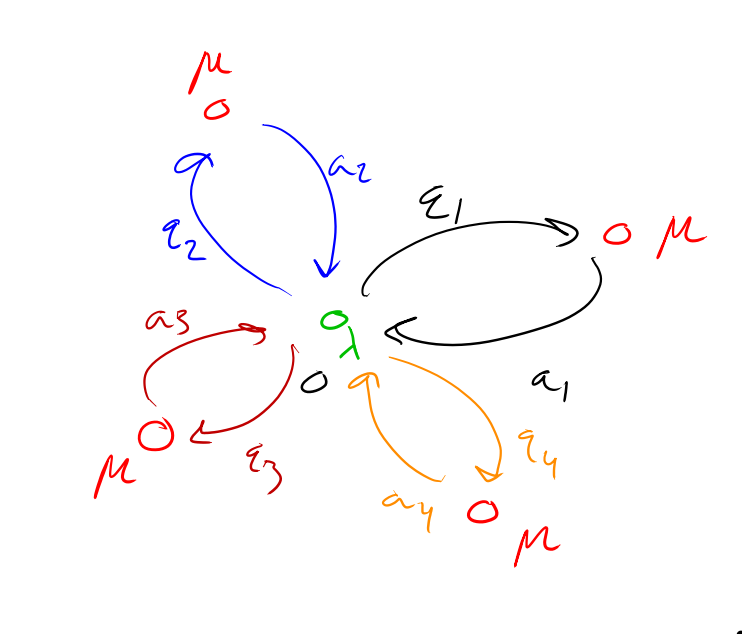# 5

Epistemological Status: I did almost no literature review and could very well be reinventing the wheel here. This does seem to give an alternative prompt strategy (permute things) for Language Models.

I'm going to ignore the specific details of GPT and focus on the idea of predicting an output token given the history up to some horizon . This is an -gram Markov model. Technically a language model predicts the unconditional probability of a sequence, but in practice, we usually do the above.

I'm interested in formalizing a particular prompt format for GPT. The class I'm interested in are structured tasks:

We can represent this pictorially,Recurrent S-tasks are an example of a sunflower. An example would be prompted addition. We have,

The main property a recurrent S-task enjoys is probability invariance under permutation,

I believe this setup is useful for getting more precise about how the language model operates. Consider an accumulation task,

Is it a recurrent task? Well, technically yes. However, it isn't feasible because we don't know what the variable total is. However, consider the modification,

Now, we have a feasible recurrent task. When we design prompts using the original we write out . What we're showing is that the invariance is not at the level, not feasible, instead, it's at the level. So we should apply the substitution rule . In general, the advantage of using recurrent tasks would be that they would be invariant under permutation which would serve as a form of regularization on the output.

New Comment